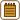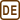## Electron flying into an electric field

An electron flies with a certain velocity in an area where two electric fields are acting simultanenously: a horizontal field Eh = 400 Vm-1 and a vertical field Ev = 300 Vm-1. The electron flies into the combined field along with its field lines and its velocity increases twice on a distance of  2.7 mm. Asses the final velocity of the electron.

• #### Two possible solutions

The task can be approached in two ways. We show both of them.

A. Studying the movement of the electron
B. Studying the laws of energy conservation

• #### Hint for both solutions

The electric field is assigned as two components. What is the total electric field?

• #### Option A – Hint

What type of motion does the electron perform after flying into the homogeneous electric field along with the field lines?

• #### Option A – Solution: Electron motion

The homogeneous electric field exerts a constant force on the electron in the direction of the field lines. As the electron flied along the field lines, the force changes only the magnitude of its velocity, not its direction. The electron thus moves along a straight line with a uniform acceleration and a non-zero initial velocity. It follows that we can limit the calculation to one dimension. The following relationships apply to this type of motion

$v=v_0 + at,$ $s=v_0 t + \frac{1}{2}at^{2}.$

Let us now calculate the final velocity vf of the electron. We start by calculating v0. Because the speed increases twice on the given distance, we can write vf = 2v0

$2v_0 = v_0 + at\qquad \Rightarrow \qquad v_0 = at.$

We now put this expression into the formula for the distance travelled:

$s=v_0t+\frac{1}{2}at^{2}= at^{2}+\frac{1}{2}at^{2}=\frac{3}{2}at^{2},$ $t=\sqrt{\frac{2s}{3a}}.$

We derived the equation for the time t, that it takes the electron to double its velocity. To determine the initial speed v0, we use the relationship v0 = at and we substitute for t

$v_0 = a \sqrt{\frac{2s}{3a}}=\sqrt{\frac{2sa}{3}}.$

We get acceleration a from the 2nd Newton’s law. For this we need the electrical force Fe exerted by the field on the electron, which is given by $$F_e=Eq$$. This relationship is one-dimensional, and q is the charge of the electron. We then get the equation for the acceleration

$m_\mathrm e a = E e\qquad \Rightarrow \qquad a=\frac{E e}{m_\mathrm e},$

where m e is the electron mass and e is the charge of the electron, which is equal to the elementary charge. We find these constants in the tables.

We substitute the acceleration into the formula for initial velocity

$v_0 = \sqrt{\frac{2sa}{3}}=\sqrt{\frac{2sE e}{3m_\mathrm e}}.$

We now determine the magnitude of the total electric field E . We use the Pythagoras theorem because the horizontal Eh and the vertical Ev components of the field are perpendicular to each other

$E = \sqrt{E^{2}_\mathrm {v}+E^{2}_\mathrm {h}}.$

According to the assignment, the final speed is twice the initial speed

$v_\mathrm f = 2v_0=2\sqrt{\frac{2sE e}{3m_\mathrm e}}=2\sqrt{\frac{2se\sqrt{E_\mathrm {v}^2+E_\mathrm {h}^2}}{3m_\mathrm e}}.$
• #### Option B – Hint

What can we say about the kinetic energy at the beginning and at the end of the motion? Was there any work done during the motion?

• #### Option B – Solution

For the kinetic energy of the electron, we have the equation

$E_\mathrm {k1}+W_\mathrm e=E_\mathrm {k2},$

E k1 is the kinetic energy of the electron when it entered the field, We is the work done by the electric force Fe on the distance s, and Ek2 is the kinetic energy of the electron at the end of the movement. The electric force acts in the direction of motion, and the work that it does thus can be calculated as $$W_\mathrm e=F_\mathrm es$$. We express the energy and work

$\frac{1}{2}m_\mathrm e{v_0}^2+ F_\mathrm es=\frac{1}{2}m_\mathrm e {v_\mathrm k}^2,$

where me is the electron mass and e is the elementary charge - we find these last two constants in the tables. We calculate the electric force Fe as $$F_\mathrm e=Ee$$.

Now, we just put in the assigned fact that the final velocity of the electron vf is double its initial velocity v0 ($$v_\mathrm f=2\,v_0$$) . We substitute and rearrange the formula

$\frac{1}{2}m_\mathrm e{v_0}^2+Ees=\frac{1}{2}m_\mathrm e {(2v_0)}^2$ $E e s = \frac{3}{2} m_\mathrm e {v_0}^2.$

We express v0 from this equation:

$v_0 = \sqrt{\frac{2E es}{3m_\mathrm e}}.$

Last, we only need to assess the magnitude of the total electric field E and then we calculate the final veloctity of the electron vf. The total field that is composed of two perpendicular components Eh and Ev is given by the Pythagorean theorem:

$E = \sqrt{E^{2}_\mathrm {v}+E^{2}_\mathrm {h}}.$

The final velocity vf is then

$v_\mathrm f = 2v_0=2\sqrt{\frac{2E es}{3m_\mathrm e}}=2\sqrt{\frac{2se\sqrt{E_\mathrm v^2+E_\mathrm h^2}}{3m_e}}.$
• #### Notation and numerical calculation

 Eh = 400 Vm-1 Horizontal electric field Ev = 300 Vm-1 Vertical electric field s = 2.7 mm = 2.7·10-3 m Displacement of the electron vk = ? (ms-1) Final velocity of the electron From the tables: e = 1.6·10-19 C Elementary charge me = 9.1·10-31 kg Electron mass

$v_\mathrm k=\sqrt{\frac{8se\sqrt{E_\mathrm v^2+E_\mathrm h^2}}{3m_\mathrm e}}= \sqrt{\frac{8{\cdot} 2.7{\cdot} 10^{-3}\cdot 1.6{\cdot} 10^{-19}\sqrt{300^2+400^2}}{3{\cdot} 9.1{\cdot} 10^{-31}}}\,\mathrm{ms^{-1}}\,\dot{=}\,8{\cdot} 10^{5}\,\mathrm{ms^{-1}}\,\dot{=}\,800\,\mathrm {km \cdot s^{-1}}$